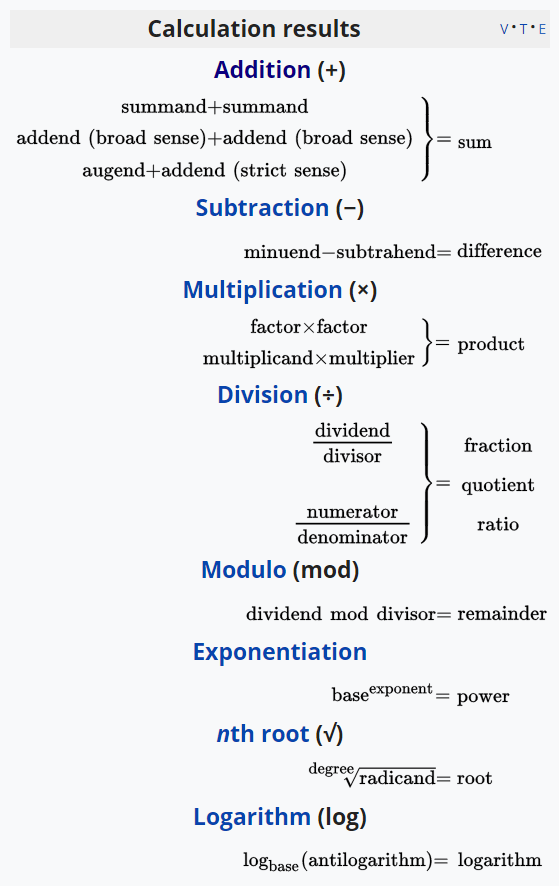## Khan Academy Math

29th November 2018 at 12:03am

## TOC

• Early math
• Arithmetic
• Pre-algebra
• Algebra I
• Geometry
• Algebra II
• Trigonometry
• Precalculus
• Statistics & probability
• Calculus
• AP Calculus AB
• AP Calculus BC
• Differential equations
• Linear algebra

## Concepts

### Early math + ArithmeticPicture from Wikipedia

• counting
• number, 2-digit number, 3-digit number
• Elementary arithmetic: addition, subtraction, multiplication, division, regrouping (carring, borrowing), times tables, basic facts, quotient, remainder
• Deci- (or places): tens, hundreds; tenth, hundredth
• Geometry: size, size length, conner; shapes, fractions of shapes, composing shapes
• Basic Facts: In Basic Facts, the arithmetic operations such as addition, subtraction, multiplication, and division are performed mainly by using the one-digit numbers (1 to 9).
• negative number, positive number, opposite number, absolute value
• fraction (To be continued from https://www.khanacademy.org/math/arithmetic-home - Fraction)

## Long Long After

• cartesian coordinate system, polar coordinate system